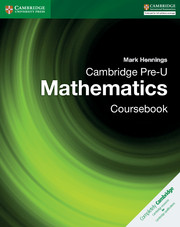Cart
Look Inside£40.00

Availability: In stock

× This is a Sterling guide price. You will be invoiced in the appropriate currency for your country.
• ISBN:9781316635759
• Format:Paperback
• Subject(s):Mathematics
• Qualification:Cambridge Pre-U
• Author(s):Mark Hennings
• Available from: June 2017

Cambridge Pre-U Mathematics offers a comprehensive resource for students to develop the thinking skills and logic required of the Cambridge Pre-U Mathematics syllabus (9794).

£40.00

Availability: In stock

× This is a Sterling guide price. You will be invoiced in the appropriate currency for your country.

Send a Query

formRow
×
• DESCRIPTION

This Cambridge Pre-U Mathematics Coursebook provides a comprehensive resource to prepare students for the high level of mathematical knowledge expected for progression through the Pre-U syllabus. The chapters have been arranged to provide logical progression through the course, and includes clear explanation of concepts, detailed worked examples and focused exercises to help practice and consolidate skills.

• Contents
• FEATURES
• Detailed exposition of new mathematical concepts, followed by worked examples to help students feel confident.

• Comprehensive coverage of the syllabus with extension materials marked for students to investigate concepts further.

• Clearly stated learning objectives to allow students to keep track of their progress.

• Inclusion of STEP questions in practice exercises with worked solutions to provide students with examples and demonstrations of this type of questioning.

• CONTENTS
• 1. Surds and indices
• 2. Coordinate geometry
• 4. The Modulus Function
• 5. Polynomials
• 6. Algebraic fractions
• 7. Functions
• 8. Circles, radians and elementary trigonometry
• 9. Sequences and series
• 10. The Binomial Theorem
• 11. Differentiation
• 12. Integration
• 13. Trigonometry
• 14. Exponentials and logarithms
• 15. Further differentiation
• 16. Differentiating implicit and parametric equations
• 17. Further integration
• 18. Differential equations
• 19. Vector geometry
• 20. Complex numbers
• 21. Numerical methods
• 22. General kinematics
• 23. Force and equilibrium
• 24. Newton's Law of Motion
• 26. Linear momentum and impulse
• 27. The motion of projectiles
• 28. Analysis of data
• 29. Correlation and regression
• 30. Permutations and combinations
• 31. Probability
• 32. Random variables
• 33. The Normal Distribution
• 34. Problem-solving techniques

Not already registered? Create an account now. ×

Other Cambridge websitesNeed a local sales consultant?